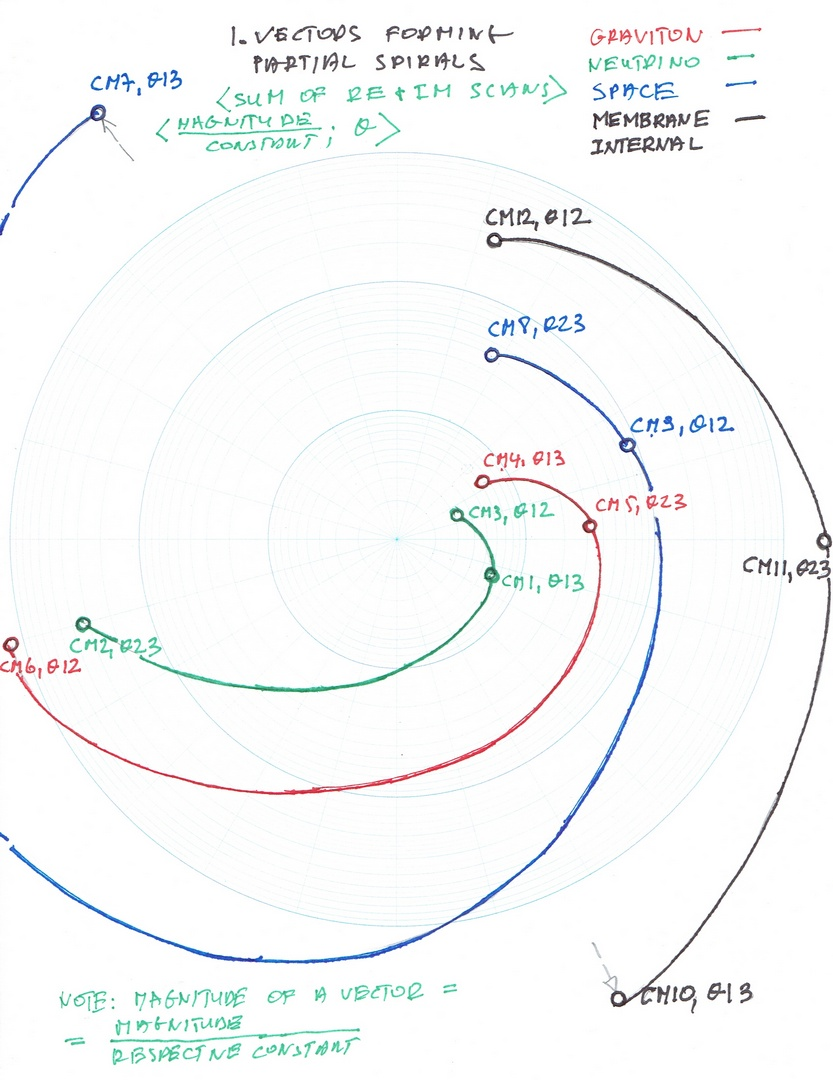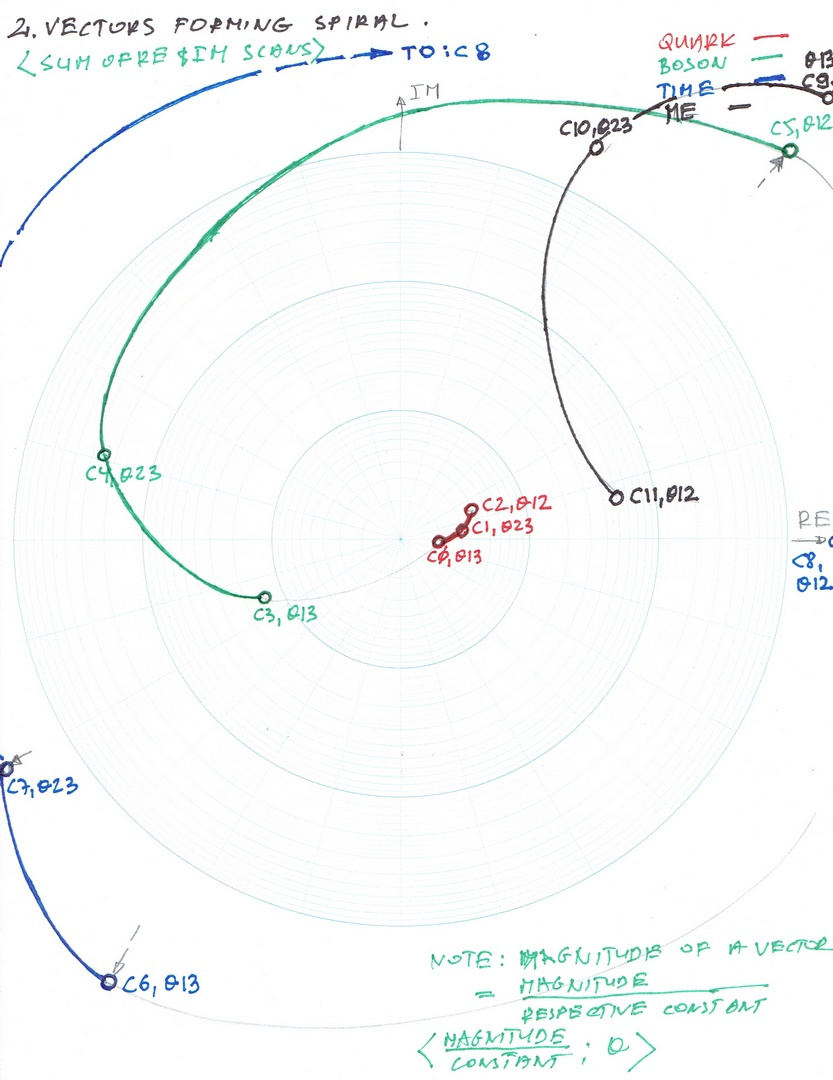2 March 2020 AD; Bl. Charles the Good (1124)

In this short article, I will present more graphs, where the output will be a function both of the magnitudes of the vector as well as the angles theta. The magnitudes and angles obviously form a complex number.

The first graph output (the sum of the Real and Imaginary Scans) is the magnitude divided by the respective constant and angle theta. Those graphs are of Graviton, Neutrino, Space and Internal Membrane. As you can see on the graph the magnitudes and angles form four different spirals and the beginnings of the spirals are almost on the straight line.The second graph (Fig 2) is similar to the Fig 1, it shows also magnitudes of the vector divided by a respective constant and angle theta for Quark, Boson, Time and External membrane. This time Quark, Boson and Time are forming a spiral and the External Membrane forms a separate spiral.Fig 3  graph shows a different relation - it shows a relation between 17 of the vectors and angles with similar (+/- 5 degrees or so) angles theta. What is interesting is the fact that when you start counting the quantity of the vectors with almost the same angles theta starting in the 3rd quadrant, either ClockWise or CounterClockWise they will form a sequence: 2, 3, 2, 3, 2, 3, 2. This is really interesting. Again, the magnitudes of the vectors are divided by a respective constant and the angles theta are not.This much for now. In the next article, the next and the last graph will be the first attempt of showing that there exists another Universe attached to ours, also consisting of 24 elements but with completely different numerical values than the elements of our Cosmos. It will also show that the one value of the Time Element is undefined, and therefore all dreams about time machine etc. are not possible*. There might be some speculations about the Parallel  Universe and so on. As you probably already figured out a long time ago, I am the Conservative Catholic and for me this another section of the Universe is just Heaven - a place of the angels, saints and of course partly of Our Lord God (Heaven can not contain Him).

*Matthew 24:36: "But concerning that day and hour no one knows, not even the angels of heaven, nor the Son, but the Father only." (I will do one day what I call "Mathematical Theology". For now, the undefined value on the constant C8 i.e. π represents God the Eternal Father, "e" - the Euler's Constant represents the Son, Jesus Christ and the ratio of "π/e" is the symbol of the Holy Spirit. "Incidentally" this ratio, this "fraction" is used to compute the Imaginary and Real Scans, giving all those values of thetas (mixing angles), coupling constants and CP Violating Phase angles (matter/antimatter) precisely.